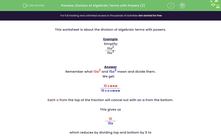# Practise Dividing Algebraic Terms with Powers

In this worksheet, students will divide algebraic terms giving their answers as a single fraction.Key stage:  KS 3

Curriculum topic:   Algebra

Curriculum subtopic:   Use and Interpret Algebraic Notation

Difficulty level:#### Worksheet Overview

This activity is about the division of algebraic terms with powers.

Example

Simplify:

 10a3 15a2

Remember what 10a3 and 15a2 mean and divide them.

We get:

 10 x a x a x a 15 x a x a

Each a from the top of the fraction will cancel out with an a from the bottom, leaving just one a the top.

However, we need not write out our method like this each time.

Remember the rules of indices for division:

a÷ an = am-n

In this example, we would calculate a3 - 2 = a1 or a

This gives us

 10a 15

which reduces by dividing the top and bottom by 5 to

 2a 3

Remember that we can use letters other than a when we write algebraic terms - and that these have to be alike to cancel each other out.

### What is EdPlace?

We're your National Curriculum aligned online education content provider helping each child succeed in English, maths and science from year 1 to GCSE. With an EdPlace account you’ll be able to track and measure progress, helping each child achieve their best. We build confidence and attainment by personalising each child’s learning at a level that suits them.

Get started